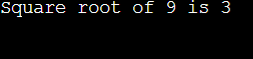# Square Root using binary search

## Introduction:

You will be given the number ‘X .’ You need to find the Square root(sqrt) of that given number.

If the given number is not a perfect square, you need to return the floor value of the Square Root i.e., the nearest smaller integer.

Let us see a few examples:-

Input: 9

Output: 3

Explanation: Square root of 9 is 3. Hence, the output is 3.

Input: 8

Output: 2

Explanation: Square root of 8 is 2.82. Since it is a decimal value, the answer will be its floor value i.e., 8.

## Approach 1:

The square root of the number X can be between 1 and X only. Hence, we can check for each value from 1 to X if it is the given number's square root.

Refer to the below implementation for the above approach.

``````class Main{

public static int mySqrt(int x) {

//It will store the square root of x
long sqrt = 0;

/*
Running a for loop
and checking every possible
value for the square root.
*/
for(long i = 1; i <= x; i++){
if(i * i <= x){
sqrt = i;
}
else {
break;
}
}

return (int)sqrt;
}
public static void main (String[] args) {
int X = 9;
System.out.println("Square root of "+X+" is "+mySqrt(X));
}
}``````

Output:Time Complexity: The time complexity for the above approach is O(sqrt(X)) because we run a for loop till the sqrt(x) only.

Space Complexity: The space complexity for the above code is O(1) because we are not using any auxiliary space.

## Approach 2:

We can apply Binary Search instead of Linear search to find the square root of a given number.

The reason behind using binary search is that the square of any number ‘N’ increases if we increase the value of ‘N’ and decreases if we decrease the value of ‘N’.

From above, we can see that it is a monotonically increasing function. Hence, we can apply binary search on it.

We will apply binary search on the Square Root of the given number ‘X’ and check if it is a potential Square Root of a given number ‘X’ or not.

Refer to the below implementation of the above approach.

``````class Main {

public static int mySqrt(int x) {

// Base Cases
if (x == 0 || x == 1){
return x;
}

// Do Binary Search for floor(sqrt(x))
long start = 1, end = x, ans = 0;

while (start <= end)
{
long mid = (start + end) / 2;

// If x is a perfect square
if (mid * mid == x){
return (int)mid;
}

/*
Since we need floor, we update answer when mid*mid is
smaller than x, and move closer to sqrt(x)
*/
if (mid * mid < x){
start = mid + 1;
ans = mid;
}

// If mid * mid is greater than x
else{
end = mid - 1;
}
}
return (int)ans;
}
public static void main (String[] args) {
int X = 9;
System.out.println("Square root of "+X+" is "+mySqrt(X));
}
}
``````

Output:Time Complexity: The time complexity for the above approach is O(log(X)) because we are doing a binary search in the range 1 - X.

Space Complexity: The space complexity for the above code is O(1) because we are not using any auxiliary space.

Have you noticed how Binary Search reduces the time complexity? Binary Search has always been one of the most asked topic in interviews, to get complete hands-on experience on various other question which can be asked on Binary Search, you should watch the below video.

## FAQs:

1. What optimization did we do on the brute force approach to solve this problem?
• We applied binary search instead of linear search because the square of any number ‘N’ increases if we increase the value of ‘N’ and decreases if we decrease the value of ‘N’. From above we can see that it is a monotonically increasing function. Hence, we can apply binary search on it.

2. What is the time complexity for the optimized approach?

• The time complexity for the optimized approach 2 is O(log(X)) because we are doing a binary search in the range 1 - X.

## Key Takeaways:

In this blog, we have covered the following things:

1. We first discussed the Brute Force approach to solve this problem.
2. Then we saw how we optimized the brute force approach by applying Binary Search instead of a Linear Search.

If you want to learn more about Dynamic Programming and want to practice some questions which require you to take your basic knowledge on Dynamic Programming a notch higher, then you can visit our Guided Path for Binary Search. To practice this problem, you can visit Practice Problem.

Until then, All the best for your future endeavors, and Keep Coding.